# The ABC's of Distances

It is almost impossible to tell the distances of objects we see in the sky. Almost, but not quite, and astronomers have developed a large variety of techniques. Here I will describe 26 of them. I will ignore the work that went into determining the astronomical unit: the scale factor for the Solar System, and just consider distances outside of the Solar System.

## A. TRIGONOMETRIC PARALLAX

This methods rates an A because it is the gold standard for astronomical distances. It is based on measuring two angles and the included side of a triangle formed by 1) the star, 2) the Earth on one side of its orbit, and 3) the Earth six months later on the other side of its orbit.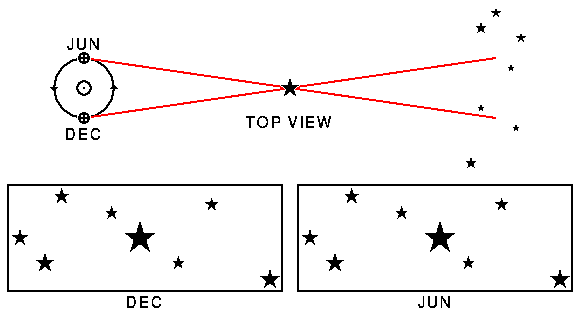The top part of the diagram above shows the Earth at two different times, and the triangle formed with a nearby star and these two positions of the Earth. The bottom part shows two pictures of the nearby star projected onto more distant stars taken from the two sides of the Earth's orbit. If you cross your eyes to merge these two pictures, you will either see the nearby star standing in front of the background in 3-D, or else get a headache.

The parallax of a star is one-half the angle at the star in the diagram above. Thus the parallax is the angle at the star in an Earth-Sun-star triangle. Since this angle is always very small, the sine and tangent of the parallax are very well approximated by the parallax angle measured in radians. Therefore the distance to a star is

```D[in cm] = [Earth-Sun distance in cm]/[parallax in radians]
```
Astronomers usually say the Earth-Sun distance is 1 astronomical unit, where 1 au = 1.5E13 cm, and measure small angles in arc-seconds. [Note that 1.5E13 is computerese for 15,000,000,000,000] One radian has 648000/pi arc-seconds. If we use these units, the unit of distance is [648000/pi] au = 3.085678E18 cm = 1 parsec. A star with a parallax of 1 arc-second has a distance of 1 parsec. No known stars have parallaxes this big. Proxima Centauri has a parallax of 0.76". [The double quote is used to denote arc-seconds (as well as inches).]

The first stellar parallax (of the star 61 Cygni) was measured by Friedrich Wilhelm Bessel (1784-1846) in 1838. Bessel is also known for the Bessel functions in mathematical physics.

## B. Moving Clusters

Not many stars are close enough to have useful trigonometric parallaxes. But when stars are in a stable star cluster whose physical size is not changing, like the Pleiades, then the apparent motions of the stars within the cluster can be used to determine the distance to the cluster.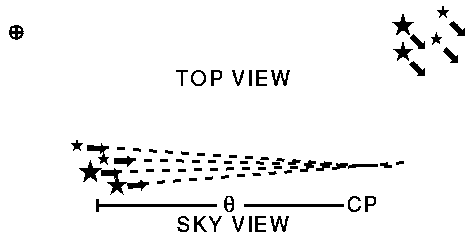The top part of the diagram above shows the space motion of a cluster of stars. Notice that the velocity vectors are parallel so the cluster is neither expanding nor contracting. But when we look at the motions of the stars projected on the sky we see them converging because of perspective effects. The angle to the convergent point is theta. If the cluster is moving towards us then the convergent point is behind the cluster but there is second convergent point on the opposite side of the sky and we use that. From the motions of the stars on the sky, known as proper motions because they are properties of individual stars, we measure theta and its rate of change, d(theta)/dt. We also need the radial velocity VR of the cluster measured using a spectrograph to see the Doppler shift. The transverse velocity, VT, (sideways motion) of the cluster can be found using VT/VR = tan(theta). The distance of the cluster is then
```D[in cm] = VT[in cm/sec]/[d(theta)/dt]

D[in pc] = (VR/4.74 km/sec)*tan(theta)/{d(theta)/dt[in "/yr]}
```
The odd constant 4.74 km/sec is one au/year. Because a time interval of 100 years can be used to measure d(theta)/dt, precise distances to nearby star clusters are possible. This method has been applied to the Hyades cluster giving a distance of 45.53 +/- 2.64 pc. The average of HIPPARCOS trigonometric parallaxes for Hyades members gives a distance of 46.34 +/- 0.27 pc (Perryman et al.).

## C. Secular Parallax

Another method can be used to measure the average distance to a set of stars, chosen to be all about the same distance from the Earth.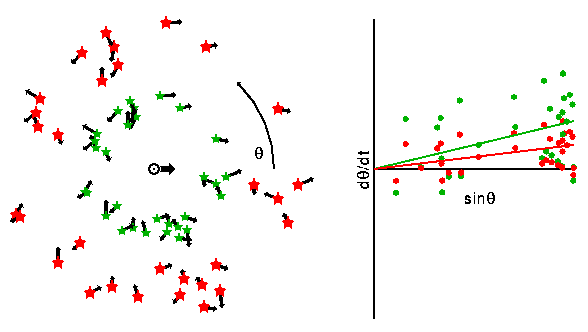The diagram above shows such a set of stars, but with two possible mean distances. The green stars show a small mean distance, while the red stars show a large mean distance. Because of the mean motion of the Solar system at 20 km/sec relative to the average of nearby stars there will be an average proper motion away from the point of the sky the Solar System is moving towards. This point is known as the apex. Let the angle to the apex be theta. Then the proper motion d(theta)/dt will have a mean component proportional to sin(theta), shown by the lines in the plot of d(theta)/dt vs sin(theta). Let the slope of this line be mu. Then the mean distance of the stars is
```D[in cm] = V(sun)[in cm/sec]/(mu [in radians/sec])

D[in pc] = 4.16/(mu [in "/yr])
```
where the odd constant 4.16 is the Solar motion in au/yr.

## D. Statistical Parallax

When the stars have measured radial velocities, then the scatter in their proper motions can be used to determine the mean distance. It is

```                  (scatter in VR)[in cm/sec]
D[in cm] = ----------------------------------------
```

## E. Kinematic Distance

The pattern of differential rotation in our galaxy can be used to determine the distance of a source when its radial velocity is known.

## F. Expansion Parallax

The distance to an expanding object like a supernova remnant like a supernova remnant such as Tycho can be determined by measuring:

1. the angular expansion rate d(theta)/dt using pictures taken many years apart, and
2. the radial velocity of expansion, VR, using the Doppler shift of lines emitted from the front and back of the expanding shell. When a spectrograph is pointed at the center of the remnant a double line is seen, with the red shifted emission coming from the back of the shell while the blue shifted emission comes from the front.

The distance is then calculated using

```D = VR/d(theta)/dt          with theta in radians
```
This method is subject to a systematic error when the velocity of the material behind the shock is less than the velocity of the shock. In supernova remnants in the adiabatic phase this is fact the case, with VR = 0.75 V(shock), so the calculated distance can be too small by 25%.

## G. Light Echo Distance

The center elliptical ring around SN1987A in the LMC appears to be due to an inclined circular ring around the progenitor. When the pulse of ultraviolet light from the supernova hit the ring, it lit up in ultraviolet emission lines which were observed by the International Ultraviolet Explorer (IUE). The first detection of these lines at time, t1, and also the time when the lines from the last part of the ring to be illuminated, t2, were both clearly evident in the IUE light curve of the UV lines. If t0 is the time that we first saw the supernova, then the extra light travel times to the front and back of the ring are:

```t1 - t0 = R(1 - sin(i))/c
t2 - t0 = R(1 + sin(i))/c
```
where R is the radius of the ring in cm. Thus
```R = c(t1-t0 + t2-t0)/2
```
When the HST was launched it took a picture of SN 1987A and saw the ring, and measured the angular radius of the ring, theta. The ratio gives the distance:
```D = R/theta           with theta in radians
```
Applied to the LMC using SN 1987A one gets D = 47 +/- 1 kpc. (Gould 1995, ApJ, 452, 189) This method is basically the expansion method applied to the expansion of the shell of supernova radiation that expands at the speed of light. It can be applied to other known geometries, as well.

## H. Spectroscopic Visual Binaries

If a binary orbit is observed both visually and spectroscopically, then both the angular size and the physical size of the orbit are known. The ratio gives the distance.

The following methods need the surface brightness of stars. The picture below shows how the surface brightness of stars depends on their colors:The colors correspond approximately to star temperatures of 5000, 6000 and 7000 K. The color shifts are quite small, but the surface brightness changes are large: in fact, I have cut the surface brightness change in half in order to make the cool star visible. By measuring the ratio of the blue flux of a star to its yellow-green flux, astronomers measure the B-V color of the star. This measure of the blue:visual flux ratio can be used to estimate the surface brightness SB of the star. Since the visual flux is measured as well, the angular radius theta of the star is known from theta = sqrt[Flux/(pi*SB)]. If the physical radius R can be found as well, the distance follows from D = R/theta with theta in radians.

The Baade-Wesselink method is applied to pulsating stars. Using the color and flux light curves, one finds the ratio of the radii at different times:

```              sqrt[Flux(t2)/SB(Color(t2)]
R(t2)/R(t1) = ---------------------------
sqrt[Flux(t1)/SB(Color(t1)]
```
Then spectra of the star throughout its pulsation period are used to find its radial velocity Vr(t). Knowing how fast the star's surface is moving, one finds R(t2)-R(t1) by adding up velocity*time during the time interval between t1 and t2. If you know both the ratio of the radii R(t2)/R(t1) from fluxes and colors and the difference in the radii R(t2)-R(t1) from spectroscopy, then you have two equations in two unknowns and it is easy to solve for the radii. With the radius and angle, the distance is found using D = R/theta.

## J. Spectroscopic Eclipsing Binaries

In a double-lined spectroscopic binary, the projected size of the orbit a*sin(i) is found from the radial velocity amplitude and the period. In an eclipsing binary, the relative radii of the stars R1/a and R2/a and the inclination of the orbit i are found by analyzing the shapes of the eclipse light curves. Using the observed fluxes and colors to get surface brightnesses, the angular radii of the stars can be estimated. R1 is found from i, a*sin(i) and R1/a; and with theta1 the distance can be found.

## K. Expanding Photosphere Method

The Baade-Wesselink method can be applied to an expanding star: the variations in radius do not have to be periodic. It has been applied to Type II supernovae, which are massive stars with a hydrogen rich envelope that explode when their cores collapse to from neutron stars. It can also be applied to Type Ia supernovae, but these objects have no hydrogen lines in their spectra. Since the surface brightness vs color law is calibrated using normal, hydrogen-rich stars, the EPM is normally used on hydrogen-rich supernovae, which are Type II. The Type II SN1987A in the Large Magellanic Cloud has been used to calibrate this distance indicator.

The following methods use the H-R diagram of stars, which gives the luminosity as a function of temperature. When the luminosity and flux of an object are known, the distance can be found using
```D = sqrt[L/(4*pi*F)]
```

## L. Main Sequence Fitting

When distances to nearby stars were found using trigonometric parallaxes in the late 19th and early 20th century, it became possible to study the luminosities of stars. Einar Hertzsprung and Henry Norris Russell both plotted stars on a chart of luminosity and temperature. Most stars fall on a single track, known as the Main Sequence, in this diagram, which is now known as the H-R diagram after Hertzsprung and Russell. Often the absolute magnitude is used instead of the luminosity, and the spectral type or color is used instead of the temperature.

When looking at a cluster of stars, the apparent magnitudes and colors of the stars form a track that is parallel to the Main Sequence, and by correctly choosing the distance, the apparent magnitudes convert to absolute magnitudes that fall on the standard Main Sequence.

## M. Spectroscopic Parallax

When the spectrum of a star is observed carefully, it is possible to determine two parameters of the star as well as the chemical abundances in the star's atmosphere. The first of these two parameters is the surface temperature of the star, which determines the spectral type in the range OBAFGKM from hottest to coolest. The hot O stars show ionized helium lines, the B stars show neutral helium lines, the A stars have strong hydrogen lines, the F and G stars have various metal lines, and the coolest K and M stars have molecular bands. The spectral classes are further subdivided with a digit, so the Sun is a G2 star.

The second parameter that can be determined is the surface gravity of the star. The higher the surface gravity, the higher the pressure in the atmosphere, and high pressure leads to line broadening and also reduces the amount of ionization in the atmosphere. The surface gravity is denoted by the luminosity class denoted by a Roman numeral from I to V with I being the lowest gravity and V being the highest (except for class VI which is sometimes seen and for white dwarfs which separately classified.) Stars with high surface gravity (class V) are called dwarfs while stars with medium gravity (class III) are called giants and stars with low gravity (class I) are called supergiants. The use of surface gravity to determine the luminosity of a star depends on three relations:

```L = 4*pi*sigma*T4*R2
L = A*Mb                      Mass-luminosity law with b = 3-4
g = G*M/R2
```
Given the temperature from the spectral type, and the surface gravity from the luminosity class, these equations can be used to find the mass and luminosity. If the luminosity and flux are known the distance follows from the inverse square law.

One warning about this method: it only works for normal stars, and any given single object might not be normal. Main sequence fitting in a cluster is much more reliable since with a large number of stars it is easy to find the normal ones.

The following methods use the properties of pulsating stars:

## N. RR Lyrae Distance

RR Lyrae stars are pulsating stars like Cepheids, but they are low mass stars with short periods (less than a day). They are seen in globular clusters, and appear to all have the same luminosity. Since the masses of RR Lyrae stars are determined by the masses of stars which are evolving off the main sequence, this constant luminosity may be caused by the age similarity in globular clusters.

## O. Cepheid Distance

Cepheid variable stars are pulsating stars, named after the brightest member of the class, Delta Cephei. These stars pulsate because the hydrogen and helium ionization zones are close to the surface of the star. This more or less fixes the temperature of the variable star, and produces an instability strip in the H-R diagram.The diagram above shows the star getting bigger and cooler, then smaller and hotter. Cepheids are brightest when they are hottest, close to the minimum size. Since all Cepheids are about the same temperature, the size of a Cepheid determines its luminosity. A large pulsating object naturally has a longer oscillation period than a small pulsating object of the same type. Thus there is a period-luminosity relationship for Cepheids. If have two Cepheids with periods that differ by a factor of two, the longer period Cepheid is approximately 2.5 times more luminous than the short period one. Since it is easy to measure the period of a variable star, Cepheids are wonderful for determining distances to galaxies. Furthermore, Cepheids are quite bright, so they can be seen in galaxies as far away as the Virgo cluster, such as M100 The only problem with Cepheids is the calibration of the period-luminosity relation, which must be done indirectly using Cepheids in the Magellanic clouds and Cepheids in star clusters with distances determined by main sequence fitting. And one has to worry that the calibration could depend on the metal abundance in the Cepheids, which is much lower in the LMC than in luminous spirals like M100.

The following methods use the properties of objects in galaxies and must be calibrated:

## P. Planetary Nebula Luminosity Function

Planetary nebulae are stars which have evolved through the red giant and asymptotic giant phases, and have ejected their remaining hydrogen envelope, which forms an ionized nebula surrounding a very hot and small central star. They emit large amounts of light in the 501 nm line of doubly ionized oxygen [O III] which makes them easy to find. The brightest planetary nebulae seem to have the same brightness in many external galaxies, so their fluxes can be used as a distance indicator. This method is correlated with the Surface Brightness Fluctuation method, which is sensitive to the asymptotic giant branch (AGB) stars before they eject their envelopes.

## Q. Brightest Stars

When a galaxy is very nearby, individual stars can be resolved. The brightness of these stars can be used to estimate the distance to the galaxy. Often people assume that there is a fixed upper limit to the brightness of stars, but this appears to be a poor assumption. Nonetheless, if a large population of bright stars is studied, a reasonable distance estimate can be made.

## R. Largest H II Region Diameters

Hot luminous stars ionize the hydrogen gas around them, producing an H II region like the Orion nebula. The diameter of the largest H II region in external galaxies has been taken as a "standard rod" that can be used to determine distances. But this appears to be a poor assumption.

## S. Surface Brightness Fluctuations

When a galaxy is too distant to allow the detection of individual stars, one can still estimate the distance using the statistical fluctuation in the number of stars in a pixel. A nearby galaxy might have 100 stars projected into each pixel of an image, while a more distant galaxy would have a larger number like 1000. The nearby galaxy would have +/- 10% fluctuations in surface brightness (1/sqrt(N)), while the more distant galaxy would have 3% fluctuations. A
figure [75 kB] to illustrate this shows a nearby dwarf galaxy, a nearby giant galaxy, and the giant galaxy at a distance such that its total flux is the same as that of the nearby dwarf. Note that the distant giant galaxy has a much smoother image than the nearby dwarf.

## T. Type Ia Supernovae

Type Ia supernovae are the explosions of white dwarf stars in binary systems. Accretion from a companion raises the mass above the maximum mass for stable white dwarfs, the Chandrasekhar limit. The white dwarf then starts to collapse, but the compression ignites explosive carbon burning leading to the total disruption of the star. The light output come primarily from energy produced by the decay of radioactive nickel and cobalt produced in the explosion. The peak luminosity is correlated with the rate of decay in the light curve. When this correction is applied, the relative luminosity of a Type Ia SN can be determined to within 20%. A few SNe Ia have been in galaxies close enough to us to allow the Hubble Space Telescope to determine absolute distances and luminosities using Cepheid variables, leading to one of the best determinations of the Hubble constant.
The following methods use the global properties of galaxies and must be calibrated:

## U. Tully-Fisher Relation

The rotational velocity of a spiral galaxy is an indicator of its luminosity. The relation is approximately
```L = Const * V(rot)4
```
Since the rotational velocity of a spiral galaxy can be measured using an optical spectrograph or radio telescopes, the luminosity can be determined. Combined with the measured flux, this luminosity gives the distance. The diagram below shows two galaxies: a giant spiral and a dwarf spiral, but the small galaxy is closer to the Earth so they both cover the same angle on the sky and have the same apparent brightness.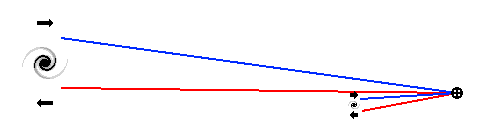But the distant galaxy has a greater rotational velocity, so the difference between the redshifted and blueshifted sides of this distant giant galaxy will be larger. Thus the relative distances of the two galaxies can be determined.

## V. Faber-Jackson Relation

The stellar velocity dispersion sigma(v) of stars in an elliptical galaxy is an indicator of its luminosity. The relation is approximately
```L = Const * sigma(v)4
```
Since the velocity dispersion of an elliptical galaxy can be measured using an optical spectrograph, the luminosity can be determined. Combined with the measured flux, this luminosity gives the distance.

## W. Brightest Cluster Galaxies

The brightest galaxy in a cluster of galaxies has been used as a standard candle. This assumption suffers from the same difficulties that plague the brightest star and largest H II region methods: rich clusters with many galaxies will probably have examples of the most luminous galaxies even though these galaxies are very rare, while less rich clusters will probably not have such luminous brightest members.
The following methods require no calibration:

## X. Gravitational Lens Time Delay

When a quasar is viewed through a gravitational lens, multiple images are seen, as shown in diagram below.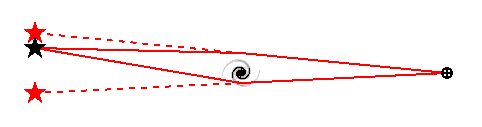The light paths from the quasar to us that form these images have different lengths that differ by approximately D*[cos(theta1)-cos(theta2)] where theta is the deflection angle and D is the distance to the quasar. Since quasars are time variable sources, we can measure the path length difference by looking for a time-shifted correlated variability in the multiple images. As of the end of 1996, this time delay has been measured in 2 quasars: the original double QSO 0957+061, giving a result of Ho = [63 +/- 12] km/sec/Mpc, and PG1115+080, giving a result of Ho = 42 km/sec/Mpc, but another analysis of the same data gives Ho = [60 +/- 17] km/sec/Mpc.

## Y. Sunyaev-Zeldovich Effect

Hot gas in clusters of galaxies distorts the spectrum of the cosmic microwave background observed through the cluster. The diagram below shows a sketch of this process. The hot electrons in the cluster of galaxies scatter a small fraction of the cosmic microwave background photons and replace them with slightly higher energy photons.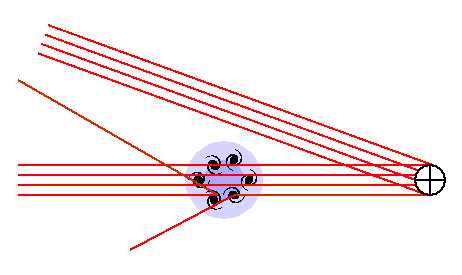The difference between the CMB seen through the cluster and the unmodified CMB seen elsewhere on the sky can be measured. Actually only about 1% of the photons passing through the cluster are scattered by the electrons in the hot ionized gas in the cluster, and these photons have their energies increased by an average of about 2%. This leads to a shortage of low energy photons of about 0.01*0.02 = 0.0002 or 0.02% which is gives a decrease in the brightness temperature of about 500 microK when looking at the cluster. At high frequencies (higher than about 218 GHz) the cluster appears brighter than the background. This effect is proportional to (1) the number density of electrons, (2) the thickness of the cluster along our line of sight, and (3) the electron temperature. The parameter that combines these factors is called the Kompaneets y parameter, with y = tau*(kT/mc2). Tau is the optical depth or the fraction of photons scattered, while (kT/mc2) is the electron temperature in units of the rest mass of the electron.

The X-ray emission, IX, from the hot gas in the cluster is proportional to (1) the square of the number density of electrons, (2) the thickness of the cluster along our line of sight, and (3) depends on the electron temperature and X-ray frequency. As a result, the ratio

```y2/IX = CONST * (Thickness along LOS) * f(T)
```
If we assume that the thickness along the LOS is the same as the diameter of the cluster, we can use the observed angular diameter to find the distance.

This technique is very difficult, and years of hard work by pioneers like Mark Birkinshaw yielded only a few distances, and values of Ho that tended to be on the low side. Recent work with close packed radio interferometers operating at 30 GHz has given precise measurements of the radio brightness decrement for 18 clusters, but only 3 of these have adequate X-ray data.

And finally:

## Z. The Hubble Law

The Doppler shift gives the redshift of a distant object which is our best indicator of its distance, but we need to know the Hubble constant.

But wait, there's MORE! Pulsar dispersion measures and interstellar extinction increase with distance along a given line of sight and can be used to determine distances. The globular cluster luminosity function can be used to estimate the distance to a galaxy from the observed brightness of its globular clusters.

Tutorial: Part 1 | Part 2 | Part 3 | Part 4
FAQ | Age | Distances | Bibliography | Relativity